Sat Oct 6 09:06:13 EDT 2018

Due Friday October 17 at 11:59 PM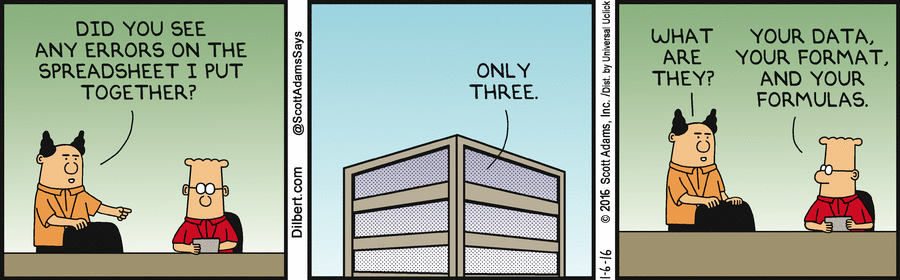The original "killer app" for personal computers was an Apple II program called VisiCalc, written in 1978 by Dan Bricklin and Bob Frankston. VisiCalc made it possible to use a computer for the kind of analyses that generations of business people had previously done by hand with paper "spreadsheets": rows and columns of related numbers that could be used to organize data and assess alternatives in a systematic way.

Today, spreadsheets are the quantitative reasoning tool for many people. In terms of market share, Microsoft's Excel is the de facto standard. Lotus 1-2-3 (the lineal descendant of VisiCalc) was discontinued by IBM in 2013. There are also free open-source spreadsheets like Gnumeric and OpenOffice Calc, and there are web-based spreadsheets like the ones from Google and Zoho. All such programs have a common computational model and similar visual appearance, and although we will use Excel in this lab, whatever you see will transfer in spirit, though not necessarily in detail, to the others. You can do this lab on Windows or a Mac.

Excel is enormously powerful and complicated, so we will investigate only a tiny fraction of its features. As you work through the specific instructions in this lab, take time to leave the official track and experiment on your own with anything that looks interesting. There's little risk in this: Excel's Undo and Redo feature let you back out of something that went wrong, or repeat the steps that got you someplace interesting. Undo and Redo are the small curved arrows near the middle of this screenshot: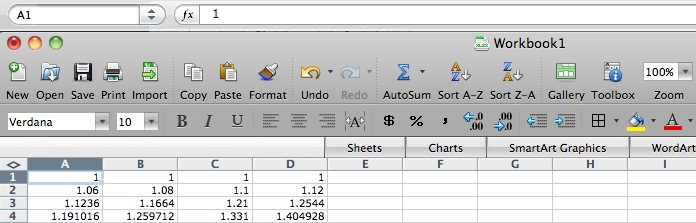If you want to know more about Excel, there are many hundreds of books, some good, and many web pages. John Walkenbach's spreadsheet page provides independent material from the author of several good books.

Part 1: Cells and Formulas
Part 2: Ranges and Functions
Part 3: Importing and Graphing Data
Part 4: How to Lie with Statistics (1)
Part 5: How to Lie with Statistics (2)

### Part 1: Cells and Formulas

#### Starting Excel

If there's an Excel shortcut on the desktop, click that; otherwise, find it with Explorer in some place like "C: | Program Files | Microsoft Office | Office11" or in Applications with Finder. When things settle down, it should look approximately like this: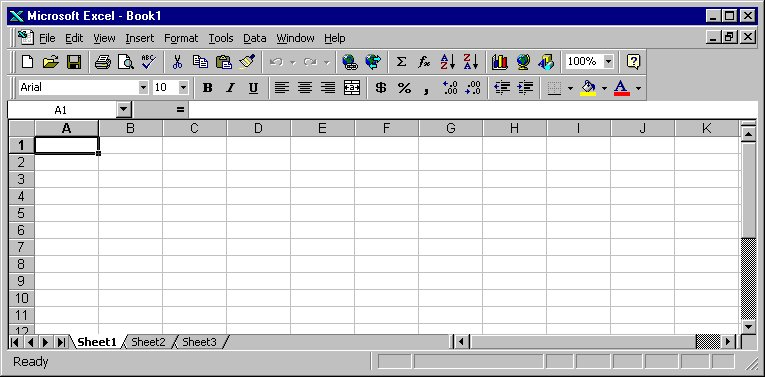Take some time now to explore the menus, toolbars, and the like.

#### Basic Concepts

The basic organizational unit in Excel is the (work)sheet, which consists of an array of rows numbered 1 through 65,536 (where does that number come from?) and columns labeled A, B, C, ..., through IV (where does IV come from?). [Excel 2007 has over 1 million rows and 16,384 columns, the latter labeled up through XFD.] Near the bottom of the Excel window you will see a tab labeled Sheet1, which is Excel's default name for the first sheet. A workbook is a collection of one or more sheets, a useful way to group related data sets in a single file but keep them cleanly separated. The default workbook name is Book1, which appears in the title bar of the window. You're going to put the results of various parts of this lab into multiple sheets, so pay attention to where things are going.

The individual elements of a spreadsheet are called cells. A cell is identified by its column (one or two letters) and row (a number); thus the cell in the upper left corner (highlighted when you started Excel) is named A1. The highlighted cell is called the active cell.

Cells can contain numbers (the most common case) or text, and their values can be set by what you type into them, loaded from files or the Internet, or computed by a formula that derives a value from the values of other cells.

Time to do some experimenting.

• Type some numbers into cells: put 1 in A1, 2 in A2, etc., down to 5 in A5.
• If you start at A1, then push Enter after each value, Excel will advance the active cell to the next row automatically.
• Experiment with correcting typing errors, going back to change a previous value, and so on. You can fix mistakes by retyping, or you can edit in the small editing window just above the column labels. The editing window and cell label are in a different place, at the top of the screen, on the Mac: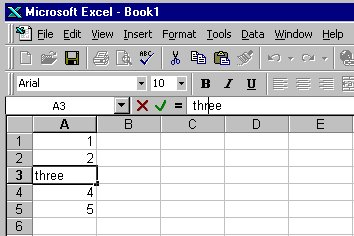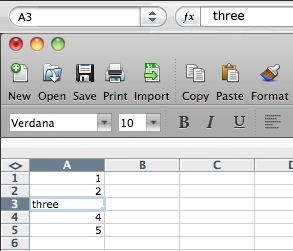You can change the format of cell data by "Format | Cells", and then select "Number" to set the numeric display, "Alignment" to control centering, "Font" to set size and color, and so on. The most common reason to adjust cell format is to cause data to be treated as numeric and displayed with the same number of significant digits, or to define the format for data that represent dates. You can instead put text in cells to serve as headings for columns or rows; text can be set in various sizes and fonts as well.

You can use commands under "Edit" to clear cell contents entirely. In all of these, you can select a single cell, a group of cells, one or more entire rows, or one or more entire columns; the action applies to the selected range.

To insert an additional row or column, highlight the row or column before which you want to insert, then use the "Insert" menu.

#### Formulas

Each cell can have attached to it a formula that computes the value displayed in the cell, usually from values in other cells. Then when cell values change, formulas are re-evaluated, and updated values are displayed.

A formula is typed into a cell just like data except that the first character must be an equals sign =. To experiment with this:

• Make sure cells A1 through A5 contain the digits 1 through 5.
• Go to cell A7 and type =A1+A2+A3+A4+A5 and push Enter. (You can type in lower case if you prefer; Excel will capitalize automatically.) If this doesn't cause cell A7 to display the value 15, check your typing.
• Now experiment with changing values in cells A1 through A5, verifying each time that the sum in A7 is properly updated.
Note that when you select A7 later, the cell value is displayed in the cell itself, but the formula is displayed in the formula area just above the sheet.

A further experiment:

• Type the formulas =A1*A1 in cell B1, =A2*A2 in cell B2, and so on through B5. Verify that the computed values are correct.
• Place the corresponding summation formula in B7 and verify that it produces the right answer.

It takes a lot of typing to enter data values and formulas this way, so Excel provides some convenient shortcuts.

• Clear all the cells that you have used so far.
• Put 1 in A1 and 2 in A2.
• Select A1 and A2.
• Place the mouse on the lower right corner of A2; the cursor should change to a little plus sign.
• Drag the plus sign down the column to row 40 and release it.
The column should fill with the integers from 1 to 40: Excel has made a (very good) guess about how you want the sequence 1, 2, ... extended and has done it for you.
• Type the formula =A1*A1 in cell B1.
• Using the same technique, extend the series to cell B40 and verify that the values are right.
Again, Excel has extended a sequence, but observe carefully that it has extended the formulas, not the values.

Finally,

• Type the formula =2^A1 in cell C1.
• Extend this series down to cell C40.
• If you narrow the column, the numbers will change format, likely twice. What's happening?
• Suppose you start with the value 2 in cell D1. What different (not the same as in C2) formula could you put in D2 and then extend to D40 that would provide the same sequence of values in D1:D40 as in C1:C40?

Excel, like Word, uses Visual Basic as a scripting language. All of Excel's myriad capabilities (including anything you can do with keyboard and mouse) are accessible from VB code. This can be used to organize much more complicated computation than would be feasible with simple formulas in cells, to tailor the interface for specific purposes, and to access all of the repertoire of other components on Windows. And of course the bad news is that spreadsheets can be just as much carriers of VB-based viruses as Word documents, so you should run Excel with macros disabled by default.

We won't pursue any of this further, but if you want to explore, VB is waiting for you: Tools / Macro / VB Editor.... One of the neatest features is that you can turn on the "macro recorder"; this will record whatever subsequent actions you perform and convert them into the equivalent VB code. It's a very effective learning tool and a valuable complement to the manual.

#### Powers of 2, Powers of 10

We've talked endlessly about how there is a reasonably close relationship between the powers of two and the powers of ten: 2^10 is a little more than 10^3, that is, 1024/1000, or 1.024. Similarly, 2^20 is more than 10^6 and the ratio is 1.049. The approximation is pretty good for a long distance though eventually it breaks down.

Your task is to make a spreadsheet that shows how good the approximation is and find the place where the ratio first becomes greater than 2.

 Clear the contents of Sheet1. Put the numbers 0, 1, 2, ..., 40 into column A. Put into column B a formula that will compute 2 raised to the power 10 times the value in column A. Put into column C a formula that will compute 10 raised to the power 3 times the value in column A. Put into column D a formula that will compute the ratio of B over C, that is, the ratio of how good or bad the approximation is. Set the cell format for column D to display exactly two digits after the decimal point. Set a yellow background color for the four cells in the row where the ratio first exceeds 2. Use "Insert | Chart" to create a graph that shows the ratio. Add a title and labels for the X and Y axes (using Toolbox | Chart Options on a Mac).

Do this with as little typing and as much use of Excel's extension feature as possible; you can probably do it by typing no more than two or three rows and then extending them. Your table should look like this when done, except that it will have more rows, more data in the graph, a title and axis labels, and a highlighted row towards the end:Notice that the approximation gets worse at worse than linear rate. To see just how fast it is getting worse, click on the chart, then select Add Trendline from the Chart menu or by right-clicking. Pick the trendline that gives the best fit to the data.

In this lab, you will be using a new sheet for each part, each with its own name. For this part,

 Double-click on the tab that says Sheet1. Type the name Power2 in its place. Save the spreadsheet in a file called lab4.xlsx.

You'll be updating this file throughout the lab, so be sure to save regularly.

### Part 2: Ranges and Functions

#### Ranges

So far we have talked about groups of cells by explicitly naming them in a summation like =A1+A2+A3, or implicitly by letting Excel extend a series for us. It's also possible to specify a rectangular array of cells in terms of the cells at the upper left and lower right corners. Such an array is called a range, and is written with the names of the two cells separated by a colon.

For example, A1:A10 describes a column range 10 cells high but only one cell wide. The range B2:K2 represents a row of 10 cells starting at B2, and A1:J10 represents an array of 100 cells, 10 by 10, that starts in the upper left corner. As a special case, A2:A2 is a range that consists of a single cell, and that can be abbreviated to the familiar A2.

Excel provides more complicated ranges, but for the most part, simple rectangular arrays are all we need. It is also possible to name a range, which is easier to understand and refer to in a big spreadsheet; we won't be using that facility here.

#### Functions

The range notation gives us a way to specify an arbitrarily large group of cells, and thus write out computations more compactly and clearly. For instance, it's impractical to type a formula like =A1+A2+A3+... if there are more than a few terms in the summation; a range is a lot easier.

Excel provides a great number of mathematical functions that perform operations over a range of cells. The simplest of these is sum, which adds up the numbers in a range: the formula =SUM(range) produces the sum of the values in the cells in the specified range.

• Go to an unused Sheet.
• Using the methods of the previous section, put the numbers 1..10 in cells A1 through A10.
• Put the formula =SUM(A1:A10) in cell A12.
• Verify that the answer is correct.
Among the other useful functions are average, median, product, max (which computes the maximum value in a range), min, and count, which counts the number of non-blank cells in the range. There are also conditionals like countif and sumif that count or sum only those cells that match some condition.
• Put numbers in some of the cells in the range A1 through C5.
• Put formulas for sum, average, max, min, and count of each row in cells E1 through E5.
• Observe how the values of the formulas are updated. Be sure you understand what is happening.

#### Inserting Rows and Columns

What happens if you need another row or column, because your data set has expanded? If you insert a row or a column within a range, Excel is pretty clever about guessing what you mean, and will extend the formula for you. But if you add a row or column at one end of the range, Excel isn't sure what you had in mind, and doesn't change the formula. Verify this behavior:

• Put the numbers 1..10 in cells A1 through A10.
• Put the formula =SUM(A1:A10) in cell A12.
• Now insert a new row 10 and put the value 100 in it. Note that the sum is now displayed in A13 instead of A12. Does the value in A13 change? Does the formula in A13 change?
• Continue the experiment by inserting a new row before row 1, and a new row after the last row of data. Check what happens to the data and the formula.
There's nothing to save for this part of the lab, but be sure that you understand how these functions work, since you will need some of them in later parts.

### Part 3: Importing and Graphing Data

#### Importing Data From Files

It's all well and good to create synthetic data to play with, but in the real world, one usually works with real numbers. In this section, you will experiment with data from Yahoo Finance, which provides a wealth of financial information in convenient formats.

The task is to generate a table that displays stock prices and relative performance for two stocks for the past two or three months. You can choose any two stocks you like; interesting pairs might be selected from among Amazon, Ebay, Google, Yahoo, Facebook, IBM, Oracle, Ford, GM, etc. Here we will just call the two stocks FOO and BAR.

• At finance.yahoo.com, select and display your first stock.
• Go to "Historical prices", set the range of dates, push "Get Prices".
• Either save the file and import it into Excel, or open it directly; the latter is easier.
• Repeat for your second stock, using the same range of dates.
You now have two Excel windows, each displaying something like this: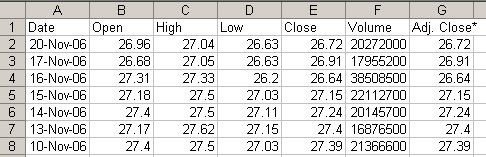Now merge the two data sequences into one sheet:

• In each sheet, delete all columns except "Adj. Close" and delete the first row. This should leave exactly one column of prices in each sheet.
• In BAR, copy column A.
• In FOO, select cell B1, then Paste; this should paste all the BAR values beside the matching FOO values.

The prices are in most recent first order, which is wrong for graphing. Reverse them:

• Insert a new column A.
• Insert numbers 1, 2, ... down column A.
• Select the three columns, then on the Data menu, pick "Sort...", and sort the data into descending order. It should now look something like this: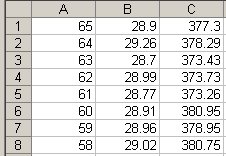At this point, if you select columns B and C, you can produce a graph that compares the two stocks, but it won't be very interesting if their prices differ by too much, as in the table above. So the next step is to make two new columns that show how the prices have changed in proportion to the first value.

• In D1, enter the formula =B1/B\$1.
• Extend it down to the end of the data.
• Repeat in E1 for column C, using the formula =C1/C\$1.
The formula =B1/B\$1 is not an error. Normally when Excel extends formulas it modifies cell references, using relative values. The \$ in a reference like B\$1 tells Excel to leave that cell reference unchanged ("absolute" instead of relative), so the subsequent formulas will be =B2/B\$1, =B3/B\$1, etc. (Try a couple of examples to see how range extension works with \$ references.) Thus each cell will contain the ratio of the price to the initial price. The result looks like this:#### Graphing Data

The next step is to draw a graph of some of this data. Excel will let you display data in a lot of different ways, some sensible and some definitely not. We want to see two graphs here. One can be a plain vanilla graph that shows the comparison between the two stocks in a simple way, like this: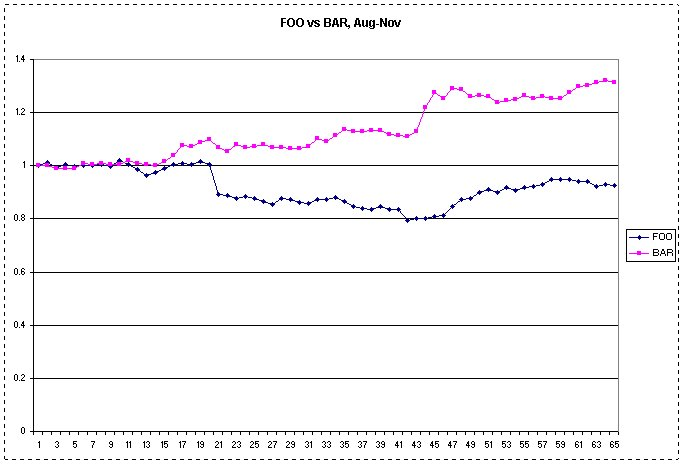Use Insert | Chart to create a graph that looks approximately like the one above but with a meaningful title, proper labels, etc. Create another graph from exactly the same data but make it as different from the previous one as you can manage. Your new graph should display the same information, and in a form that can potentially be understood. Place it near the other chart. Make the two charts approximately the same size and position them so the charts and the numeric data can all be seen at once.

Now transfer the data and the graphs to your lab4.xlsx.

 Select Edit, then Move or Copy Sheet, then "To book" lab4.xlsx. Check "Create a copy", push OK. In your lab4.xlsx, double-click on the tab for the copied worksheet and rename it Stocks.

### Part 4: How to Lie with Statistics (1)

#### Gee-Whiz Graphs

Every week, the New York Times business section shows the week's performance for 8 selected stocks; the graphical component shows how their prices have risen and/or fallen during the week. Here's the picture for a particular week long ago: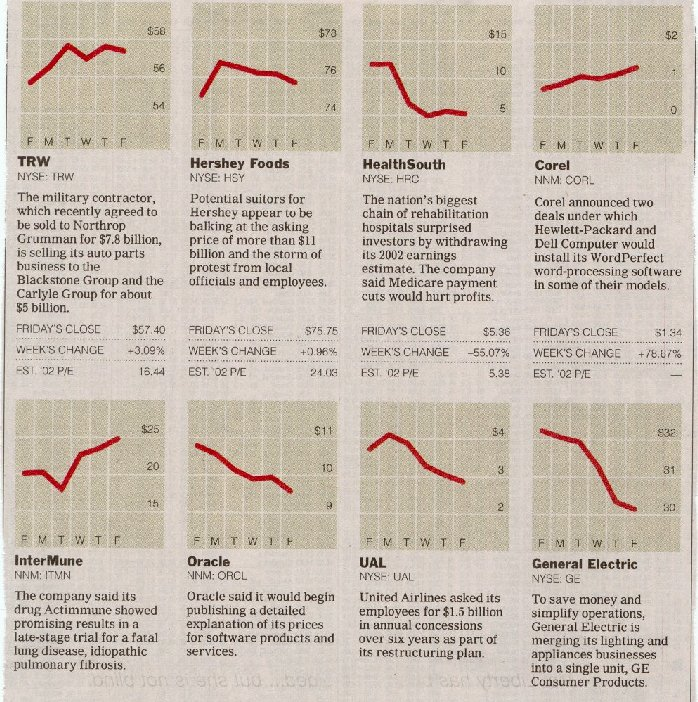A quick glance at these graphs suggests that TRW, Corel, and InterMune all went up about the same amount, for example, while HealthSouth, Oracle and UAL all went down about the same amount; GE fared a little worse.

Or did they? These are examples of what Darrell Huff, in the wonderful book How to Lie with Statistics, calls the Gee-Whiz Graph, a form of statistical chicanery that is all too common in newspapers (even the Prince!) and magazines. (Six months later, the Times printed an article decrying the practice, though without acknowledging how often they did it themselves; their subsequent charts have on average been much better.)

Gee-whiz graphs are deceptive because they use the entire chart area to give the impression of a big change. This gives entirely the wrong impression when not much is happening and it makes comparisons quite misleading. Consider TRW versus Corel. TRW seems to have risen a bit more than Corel, at least graphically, but in fact their fortunes were enormously different: TRW rose a modest 3%, while Corel went up by 78%! Similarly, one could easily conclude that GE went down more than HealthSouth, but in fact, the declines are 6.5% and 55%. (This was about the beginning of a decline for HealthSouth as more and more fraud was uncovered in their accounting.)

The heart of the deception is plotting a graph that doesn't include the full range of data. Each graph should be plotted with the Y axis beginning at zero; that would give a much more accurate sense of the magnitude of the change. And then if each is plotted with a comparable upper bound, somewhat above the largest data value, that makes it possible to compare the two graphs in a meaningful way.

Your task is to produce two sensible graphs that permit such a fair comparison, by having the Y axis start at 0 and the values just about fill the vertical range, like this graph of the Corel data: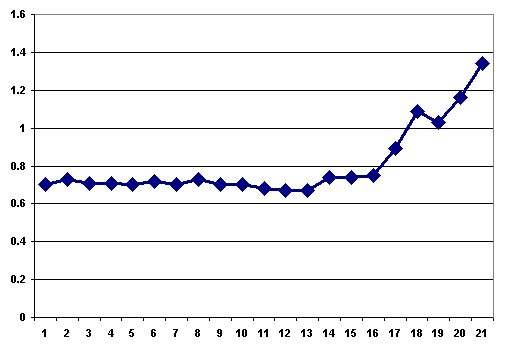The files hrc.txt and ge.txt contain the raw data from which these graphs were produced, for the entire month of August 2002. Use the closing prices from those files to produce two graphs of about the same size (2 or 3 inches on a side) but where the scale goes from 0 to the next round number above the higest value; plot them side by side, along with the data values in three columns (date, HealthSouth, GE). You're welcome to pick any other pair of interesting stocks over any period.

Note that the data in these text files is in the wrong order; use Excel's sorting capabilities (e.g., Data | Sort...) to get it into the right order.

 Go to a new sheet and rename it Lies Load the date and closing-price data from hrc.txt into A1:B22 of sheet Lies. Cut and paste is easiest but be sure to paste as text. Draw a graph approximately like the one above, but with the Y origin set to zero. Load the data from ge.txt into C1:C22 of sheet Lies. Draw a graph like the one above, with Y origin set to zero. Position the graphs side by side and near the 3 data columns.

### Part 5: How to Lie with Statistics (2)

It's always nice when others recognize one's true greatness, as US News and World Report has done every year since 2000. Lamentably they made an error for the 2009 rankings that came out in August 2008, but this was corrected in 2010 and beyond; here's the top of the list for fall 2018:But how are these rankings really determined? And just how much do they really mean?

US News reveals a bit about their methodology; basically, they collect data on a variety of factors for each school, weight the factors according to how important they seem, and then sort the results. For example, "undergraduate academic reputation" accounts for 22.5% of the score, "graduation and retention rates" for 22.5%, and alumni giving percentage for 5% (where Princeton is sure to win big).

There are at least two problems with these ranking schemes: the data itself is suspect, and the weighting factors are arbitrary. (We pass over how schools themselves might try to game the system, a tactic that is not unheard of.) In this lab, we'll accept the data values, however flaky they might be, and focus on the weighting factors.

The file usnews.xls contains some carefully fiddled data and a set of weights, loosely based on data from a few years ago, that almost preserve the original ordering; many factors have been unceremoniously dropped and a few data values have been adjusted, so don't read anything into this, especially not about the merits of individual schools then or now. Here is the display for PC and Mac: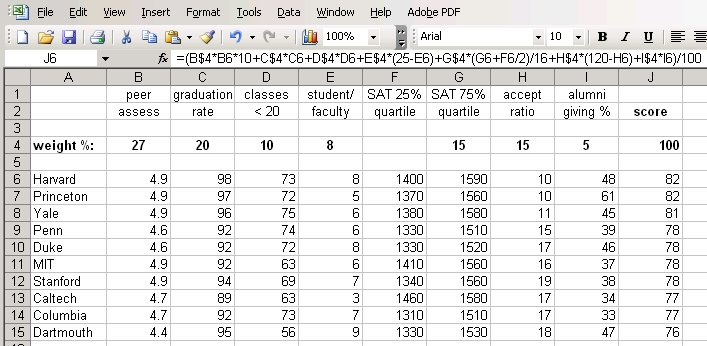The first two rows show the factors, and the fourth row gives weights that sum to 100% (cell J4). The range J6:J15 shows the computed scores. The formula box shows the formula being used to compute J4; subsequent rows have the same formula except for cell references. A couple of factors are combined (SAT) or complemented (acceptance ratio, since a low acceptance ratio is deemed better than a high acceptance ratio.

Your task is to find several sets of non-negative weights that will rearrange the schools in various ways. Note that the weight percentages in B4:I4 must sum to 100.

• Download file usnews.xls from the browser and open it in Excel. (It will appear in a new workbook.)
• Select and Copy all its cells.
• Go to Book1.
• Insert a new Sheet, and rename it Rank.
• Select cell A1 and Paste.
Now you can begin experimenting to find interesting weighting factors.

 Find a set of weights that drops Harvard as far down as you can while ensuring that Princeton is in first place. Copy the weights into B17:I17. In the interests of fairness, find a set of weights that drops Princeton as far down as you can while putting Harvard in first place. Copy them into B18:I18. Find a set of weights that raises Dartmouth as high up as you can manage. Copy them into B19:I19.

Give this a decent effort, not just the first obvious thing that happens to make a change. You should also experiment with Excel's sorting capabilities here; at the end, you should be able to sort the schools by any combination of factors, for example, by decreasing reputation score and within that by increasing acceptance rate.

### Part 6: Submitting your Work

At this point you should have a workbook with four sheets: Power2, Stocks, Lies, Rank. Check through them to make sure they look right.

• Be sure to save your work as lab4.xlsx somewhere safe. This is your backup in case something goes wrong with the submission or your computer. You could mail a copy of lab4.xlsx to yourself, as another backup. Make sure that it arrives OK, that the attachment is about the right size, etc.

When everything is working, then

Upload lab4.xlsx to the CS dropbox for Lab 4: https://dropbox.cs.princeton.edu/COS109_F2018/Lab4.